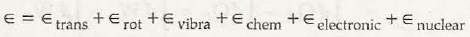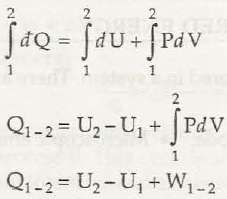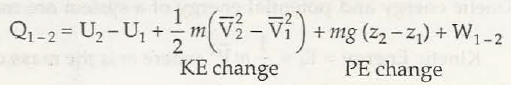There are different types of energy and the two important forms are:

1. Kinetic energy:

It is the energy that may move in the objects or mass. Wind energy is the best example of kinetic energy. The molecules of gas found in air and may move among the objects in form of kinetic energy.

1. Potential energy:

It is also a form of energy that helps to store potential so that can be used for future. For instance, water that is stored in dam for any hydroelectricity generation that is available in form of potential energy.

There are two different modes where the energy gets stored in system:

• Macroscopic energy mode
• Microscopic energy mode

Macroscopic energy mode: It is known to be the kinetic energy as well as potential energy of system that is considered. Suppose, the fluid element of mass m and centre of mass velocity is denoted by V. the macroscopic kinetic energy Ek of the fluid element is,
Ek = ½ mV2

In case fluid gets elevated to a specific height h, the macroscopic potential energy is denoted by Ep of the element,Ep = mgh which is potential energy.

The microscopic energy mode may contain energy that is stored in molecular as well as atomic structure of system. It is the energy that may consists of any molecular translational kinetic energy, chemic energy, nuclear energy, vibration kinetic energy and rotational kinetic energy.

E is represented as the energy found in a molecule and it is symbolized as:

ε = εtrans + εrot + εvib + εchem + εelectronic + εnuclearIf N is said to be the total amount of molecules, then the total microscopic energy will turn out to be:
U = NεThe total energy of system will be:E = Ek + Ep + U

Links of Next Mechanical Engineering Topics:-### Customer Reviews

My Homework Help
Rated 5.0 out of 5 based on 510 customer reviews at